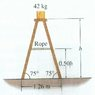# Tension in the string rope

logearav

## Homework Statement

1) A box of mass 42kg sits on the top of a ladder. Neglecting the weight of the ladder, find the tension in the rope. Assume the rope exerts horizontal forces on the ladder at each end.

m1g – T = m1a

## The Attempt at a Solution

T = m1g - m1a
This is the formula i know for calculating the tension
I have mass has 42 kg. But what is the value for acceleration here? Also I don't know because i have values for h and θ in the diagram.

## The Attempt at a Solution

#### Attachments

•s.jpg
13.2 KB · Views: 554